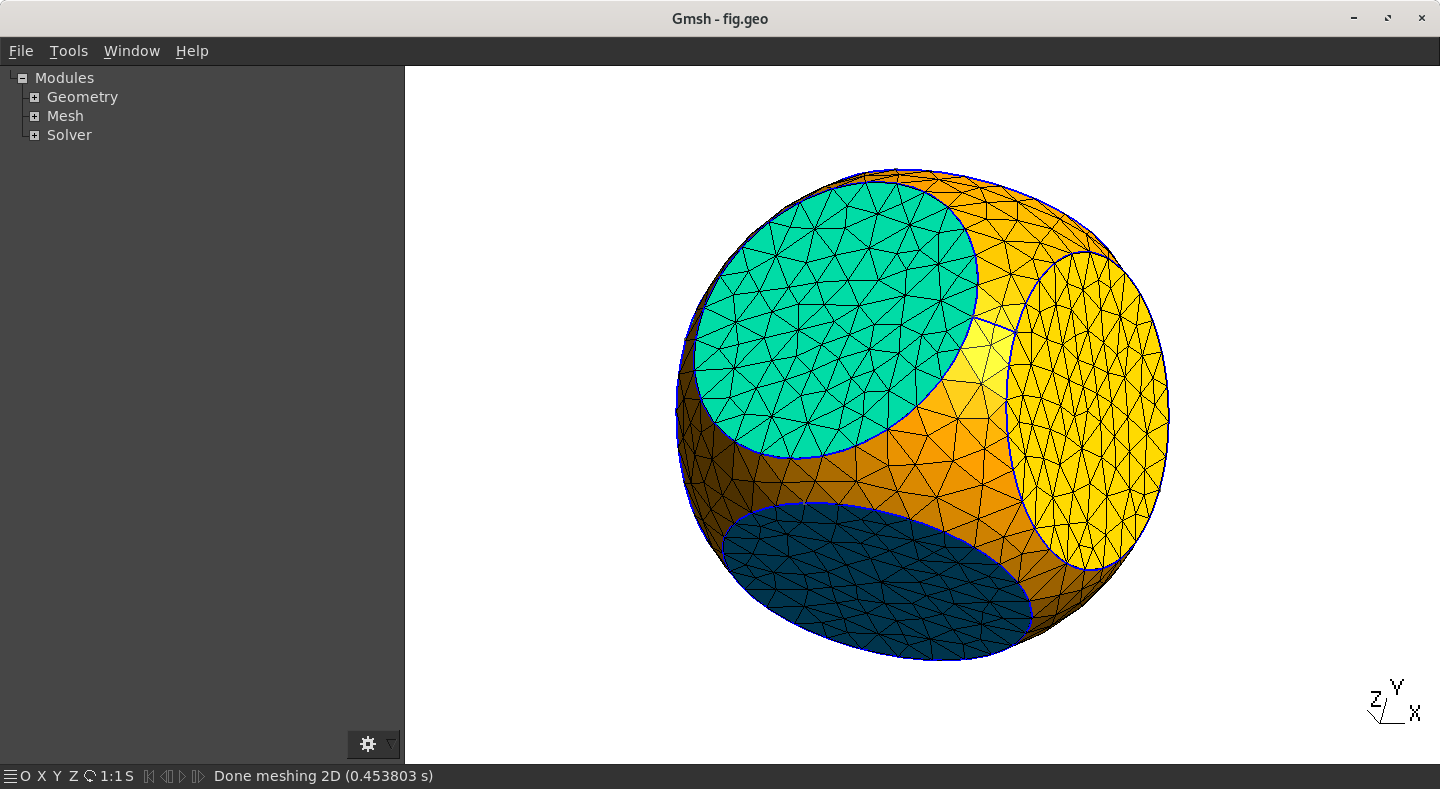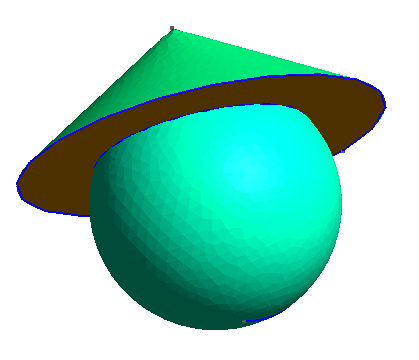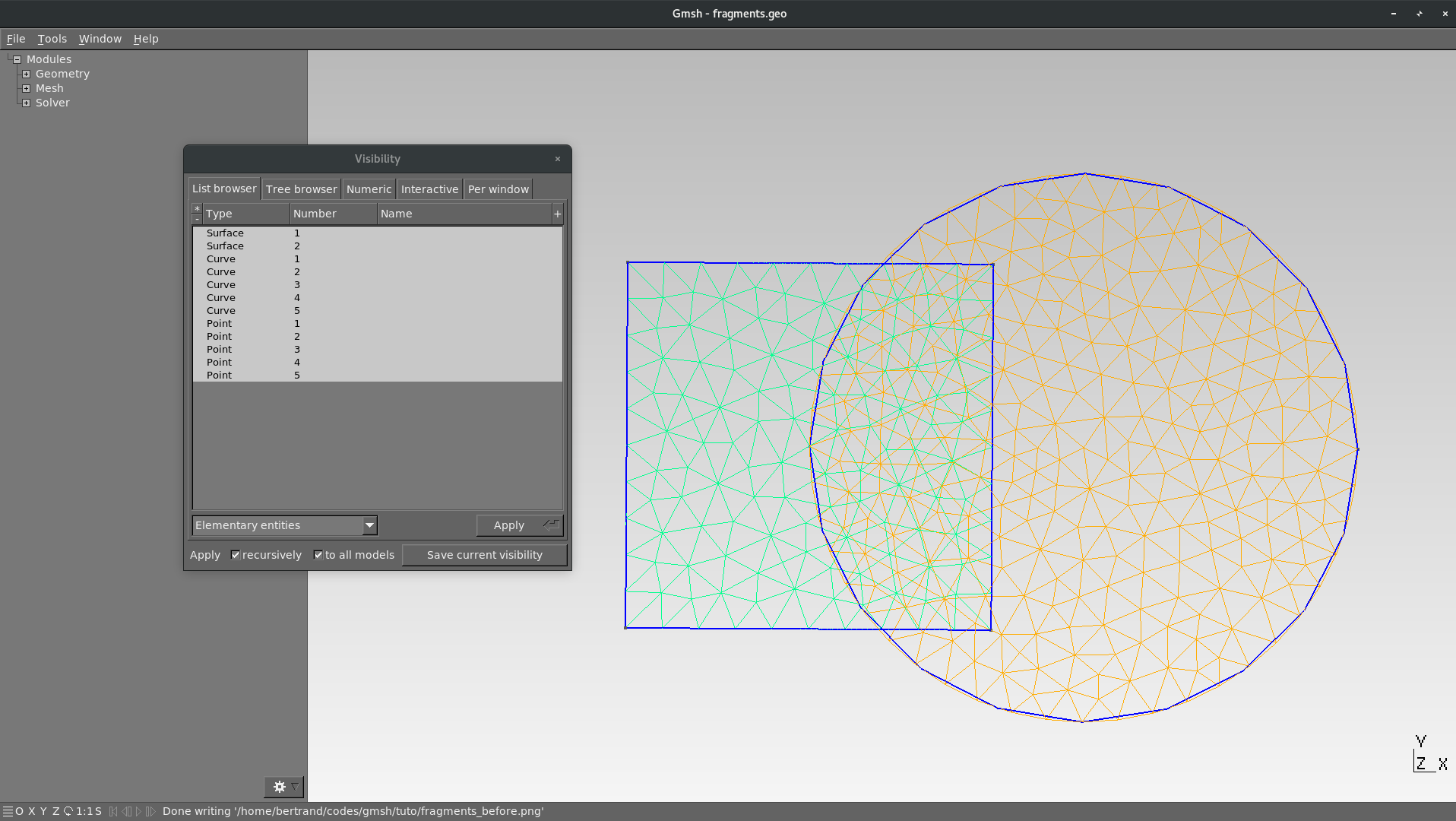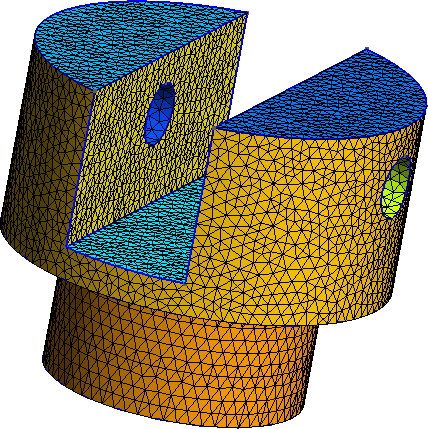# Geometries & Operations

## Geometries

### Example of a `Box`

Open a new file, `box.geo`, and copy/paste the following line on top of the file:

``````  SetFactory("OpenCASCADE");
``````

This command forces GMSH to use OpenCascade engine instead of the native one.

With this engine, you generally do not have to build the `Point` by hand, as ready-to-use geometries are provided. The mesh size must however still be set on every `Point`. A handy way to set the same mesh size to every `Point` is to use the two commands `Mesh.CharacteristicLengthMin` and `Mesh.CharacteristicLengthMax` at the beginning of the file :

``````SetFactory("OpenCASCADE");

h = 0.1; // or whatever
Mesh.CharacteristicLengthMin = h;
Mesh.CharacteristicLengthMax = h;
``````

Now, we are going to build the box. Instead of creating the `Point`, `Line`, `Surface` and finally the `Volume`, add the following line to the file

``````Box(1) = {0, 0, 0, 1, 1, 1};
``````

And here you go (⌐■_■) ! A nice box of size 1 in every direction (e.g a cube), in a single line!

The `Box` commands have the following syntax:

``````Box(index) = {x, y, z, lx, ly, lz};
``````

where

• `index`: unique identifier of the resulting elementary `Volume`
• `x`,`y`,`z`: cartesian coordinates of the “bottom left” point
• `lx`,`ly`,`lz`: size in the (resp.) x-,y- and z- direction of the box

Time to play!

1. Build a box with `lx` = 1, `ly` = 0.5 and `lz` = 0.75
2. Mesh in 2D
Using the GUI, you can check the orientation of the normal vector on the surface: `Tools→Option→Mesh` then select a value (e.g 50) at `Normals` (bottom left).

### Some available geometries

Dim. Name and syntax Description
2D `Disk(index)= {x,y,z,R}` Disk of radius `R` and center (`x`,`y`,`z`)
2D `Rectangle(index)= {x,y,z,lx,ly}` Rectangle in the (x,y) plan which the “bottom left” point is located at (`x`,`y`,`z`). The rectangle sizes are `lx` and `ly` in the x- and y-direction respectively
3D `Sphere(index)= {x,y,z,R}` Sphere of radius `R` and of center (`x`,`y`,`z`)
3D `Cylinder(index)= {x,y,z, xv, yv, zv, R, alpha}` Cylinder of radius `R` build from a circule of center (`x`,`y`,`z`), extruded along the vector (`xv`,`yv`,`zv`) . Optional parameter `alpha` represents angular opening of the Cylinder (for partial disk)
3D `Torus(index)= {x,y,z,R1, R2}` Torus of radii `R1` and `R2` and of center (`x`,`y`,`z`)
3D `Cone(index)= {x,y,z, dx,dy,dz,R1, R2}` Cone of center (`x`,`y`,`z`) , of direction (`dx`,`dy`,`dz`) and of radii `R1` and `R2` (which can be zero!)

## Boolean operations: CAD like a boss (⌐■_■)

### General Syntax

Four boolean operations are available in GMSH. They all need two input arguments: an `object` (first argument) and a `tool` (second argument). The index of the created element can be provided by the user but remember that the result can be “different” from what expected: a boolean operation can create multiple entities and hence, applying a single index does not make sense!

``````//Two possible syntax (beware the ";")
BooleanOperation{Object_List; Delete; }{Tool_List; Delete;}
BooleanOperation(index) = {Object_List; Delete; }{Tool_List; Delete;} ;
``````

The `Object_List` and `Tool_List` are not list in the programming point of view rather that an enumeration of entities (`Point, Line, Surface, Volume`). For example, the list of `Line` numbered 2, 3 and 6, `Surface` of every number from 42 to 50 and `Volume` 11 and 15 will be written as:

``````Line{2,3,6}; Surface{42:50}; Volume{11,15};
``````
`Delete;` is optional but generally recommended and forces GMSH to delete the entities passed as argument (both the `tool` and the `object`). Otherwise, there is a high risk of getting dupplicated entites!

### The 4 operations

Name and syntax Description
`BooleanIntersection` Intersection between the `objet` et l'`outil`
`BooleanUnion` Union between the `object` and the `tool`
`BooleanDifference` Substracting the `tool` to the `object`
`BooleanFragments` Compute each fragments resulting from the intersection of the entities of the `object` and of the `tool`. This command moreover delete any dupplicated entities and make every interface great again unique
`BooleanFragments` is probably the most usefull and powerful operation.

## Example: it’s a good day to dice

### Code and expectation

There is nothing better as an example to understand a tool. Let’s build a 6-faces dice in a `dice.geo` file (copy/paste the following code):

``````SetFactory('OpenCASCADE');
Mesh.CharacteristicLengthMax = 0.1;
Mesh.CharacteristicLengthMin = 0.1;
Box(1) = {-0.5,-0.5,-0.5, 1, 1, 1};
Sphere(2) = {0,0,0, 0.65};
BooleanIntersection{ Volume{1}; Delete;}{ Volume{2}; Delete;}
``````Dice

### Analyse of the code

First, the OpenCascade is set (line 1), then the cube is created (`Box`) and a sphere (`Sphere`). The associated `Volume` indices are respectively 1 and 2. Finally, the boolean operation is launch

``````BooleanIntersection{ Volume{1}; Delete;}{ Volume{2}; Delete;}
``````

The intersection between `Volume{1}` (the cube) and `Volume{2}`(the sphere) is computed. Both `Volume` are then deleted due to `Delete;` but GMSH will create a new `Volume` and the possible `Surface` and `Line` associated and, obviously, will provide them a unique identifier. The indices are generally chosen as the “first number available”. As the `Volume` 1 and 2 has been deleted, there is “high chance” that the index of the newly created `Volume` will be 1 (because “1” has been freed). This can be check by going in the menu, `Tools→Visiblity`, and check the elementary entities indices (see remark below).

Huge Warning. Numbering has been changed! You have to be very careful with that! `Line`, `Surface` and `Volume` indices are modified by the `Delete` in the boolean operations!
It can be necessary to manually check the indices of the newly created entities in `Tools→Visiblity` menu (shortcut: `ctrl + shift + v`). Chose then to display `Elementary Entities` in the dropdown menu at bottom left.
Try it without the `Delete;` command and have a look in the visualisation window (`Tools→Visiblity`). You should see multiple `Volume` that overlap each other…

Draw this head with a conical hat as in the following figure. Be sure that, in the end, there is only one `Volume`.

To help you design this nice geometry: the sphere is centered at (0,0,0) with a radius equal to 1 while the cone is of parameter (0, 0, 0.5, 0, 0, 1, 1.5, 0).Head with a conical hat

## A focus on `BooleanFragments`

Probably the most usefull but the less intuitive operation, `BooleanFragments`, does the following two operations:

1. Fragment the elementary entities: each intersection between entities are computed and become a newly entity with its own index
2. Delete every dupplicated entity, in particular common boundaries (e.g between two `Surface` or `Volume`)

Let us take the simple case of a geometry composed by a square and a disk with non empty intersection:

``````SetFactory("OpenCASCADE");
Mesh.CharacteristicLengthMin = 0.1;
Mesh.CharacteristicLengthMax = 0.1;
Rectangle(1) = {-0.5,-0.5, 0, 1, 1};
Disk(2) = {0.75, 0, 0, 0.75, 0.75};
Mesh 2; // Force GMSH to mesh
``````

Opening this file in GMSH is a deceiving experience: there are 2 meshes and they are overlapping each other!Without BooleanFragments: overlapping meshes

Let us now add the fragmentation:

``````SetFactory("OpenCASCADE");
Mesh.CharacteristicLengthMin = 0.1;
Mesh.CharacteristicLengthMax = 0.1;
Rectangle(1) = {-0.5,-0.5, 0, 1, 1};
Disk(2) = {0.75, 0, 0, 0.75, 0.75};
BooleanFragments{ Surface{1,2}; Delete; }{}
Mesh 2; // Force GMSH to mesh
``````

Three entities are created (instead of only one) but there is only one (connected) mesh and no dupplicated boundaries. The three `Surface` can be regrouped lated into a single `Physical Tag` so this is absolutely not a problem.Maillage superposé : après BooleanFragments
Arguments order and placement in `BooleanFragments` has no really impact on the result. The `tool` argument can for example be empty as above.

## Training examples

Create a nice mug as the below picture. You can use the `Rotation` operation to rotate the handle:

``````Rotate{{x,y,z}, {xp,yp,zp}, a}{List}
``````

where (`x`,`y`,`z`) is a vector on the rotation axis, (`xp`,`yp`,`zp`) a point on the axis and `a` the angle of rotation (in rad). The `List` argument contains the entities to be modified (`Line`, `Surface` and `Volume`). The quantity π in GMSH is given by the hard coded command `Pi`.Mug

Try and reproduce the following geometryMecanichal piece